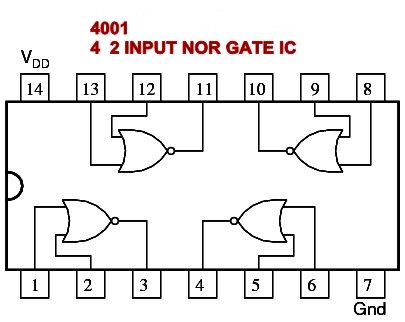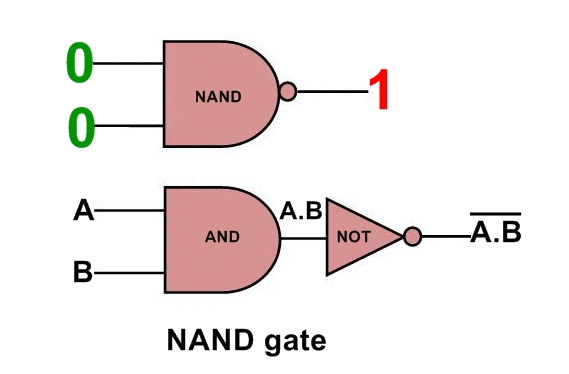### LOGIC GATES

Logic gates are the basic building elements of any digital systems or circuits. The name Logic gate is derived from the sense of the making decisions ability of such a device, and after making decisions it produce one output result. We  can say that Logic gates are the fundamental building blocks of any digital circuits or digital systems.

There are 3 basic Types of Logic gates – (1)-AND ,  (2)-OR ,  (3)-NOT

Basically Logic gates are elementary electronic logic circuit that can make a variety of different types of circuits by interconnection of these three gates to  perform complex logically operations of any computer. This is called logic design. Logic gates made a number of electronic devices and other digital components. In practical application of logic gates we see in the form of different Ics.  These Ics are in LSI (Large scale integration), VLSI (Very large scale integration ) and SSI (Small scale integration) . Input and Outputs of Logic gates in only two levels called TRUE and FALSE , or HIGH and LOW, or ON and OFF, or  very popular 0 and 1.

High (1 or TRUE or ON) means +5v

LOW(0 or FALSE or OFF) means 0v (or -ve supply)

These 2 level logic also called Positive and Negative Logic.

### AND GATE

AND gate has two or more than two inputs and only one Output. In AND gate Output is High or 1 only when each input of it has in HIGH state. Means output is 1 if only all inputs are at 1 level. If any one input goes at 0 level then the  output of AND gate becomes 0 .

Logic Symbol

Input Variables are represented by A,B,C…… and the the output is written as X. In the Boolean expression it can be written as X=A.B.C……. It Can be read as X is equal to A and B and C ….or X is equal to A dot B dot C…. or X is equal to ABC…..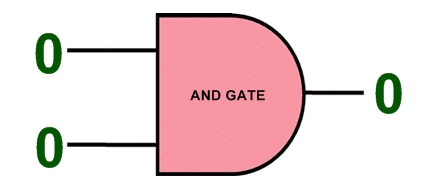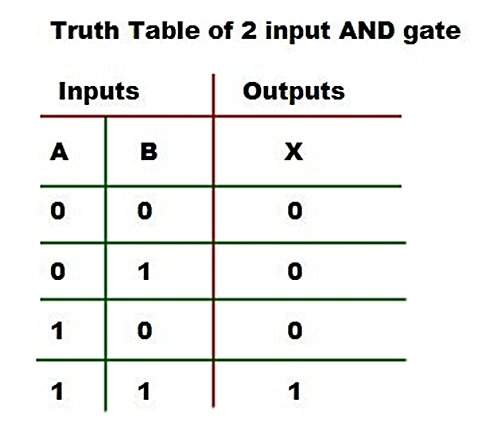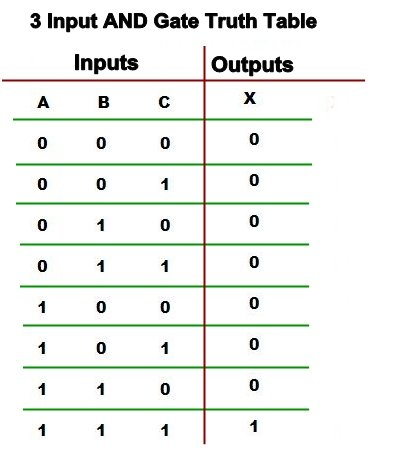### Realization of AND Gate-

AND gate may be realized by the using of diode and transistors. If it made by using diodes then it called as DL(Diode Logic) and if using transistors it called as RTL (Resistor Transistor Logic)    .

In the Diode Logic AND gate When the input A = +5v and input B is also +5v, in this case both diodes D1 and D2 are off (because of reverse mode). And therefore no current flows through resistor R. So no voltage drop occurs across R and voltage remain at the output is HIGH.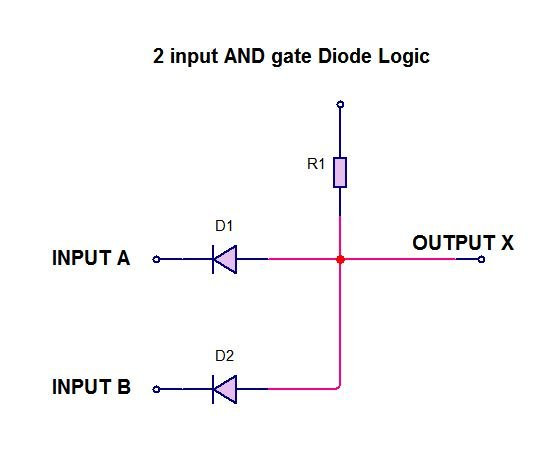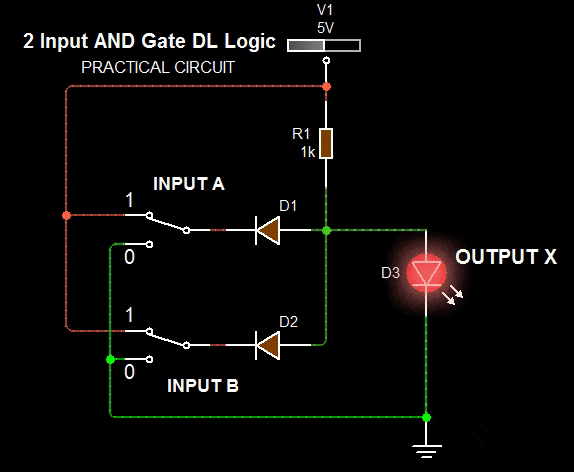When A=0, and B=0v or any one of A or B is 0v. Then the both diodes  or corresponding Diode D1 or D2 are ON and the circuit is act as short circuit. In this condition the output X=LOW or 0v.

### RTL AND Gate circuit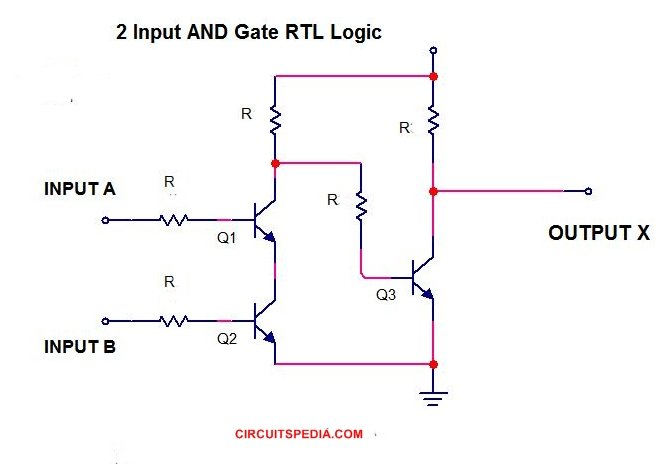In the RTL AND gate or transistor gate, When A=0v and B=0v. Then the transistors Q1 and Q2 are off but transistor Q3 remain in ON, and the voltage at  output is LOW or 0v because of Q3 ON and current pass through Q3 and voltage is dropped through R.

If Any one of input A or B is high then Either Q1 or Either Q2 will off and then no voltage drop occurs at R. So Q3 Will remain in turn on and therefore output will LOW.

If input A and input B both are HIGH then both transistors Q1 and Q2 will turn on and current passes through these transistors between ground and +5v and voltage will LOW at the collector pin of T1 and at input of Q3 . So Q3 will off and no voltage drop at collector pin and output will HIGH.  At HIGH condition the voltage at output is approx  5v (X≈5v).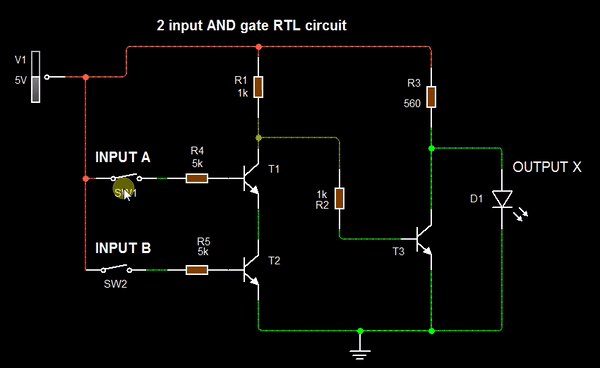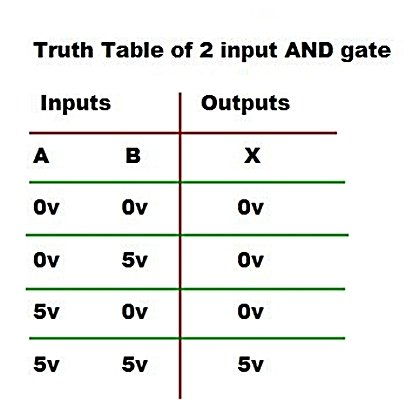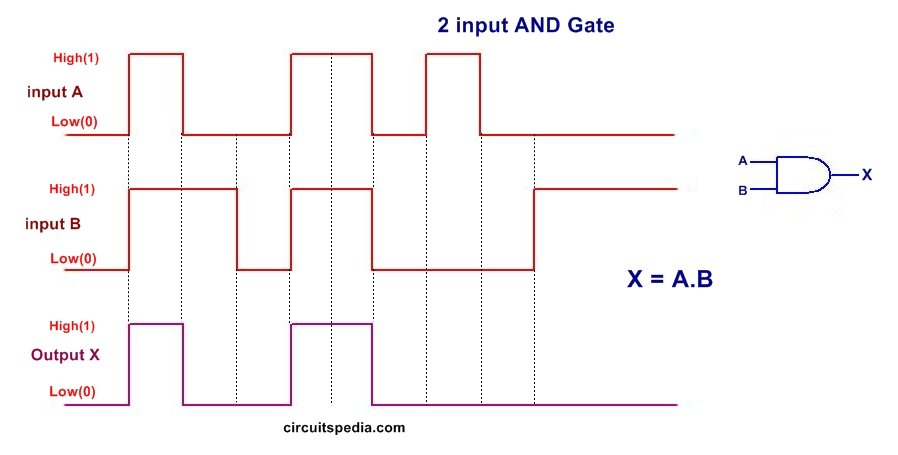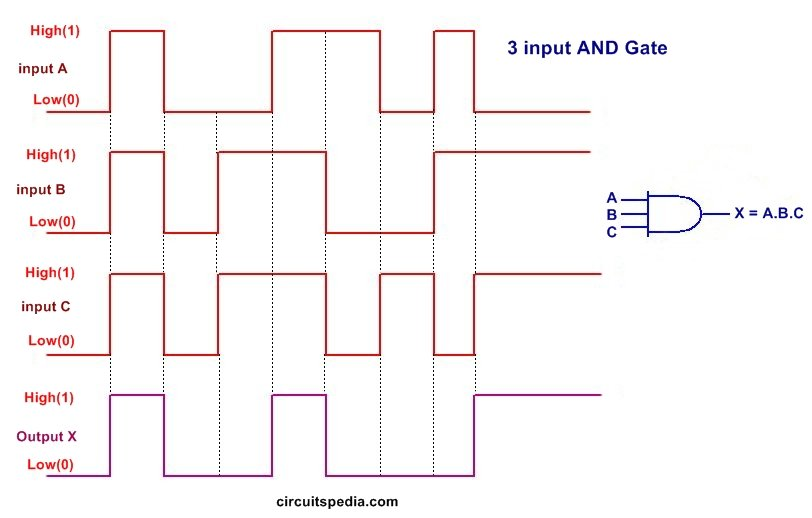### OR gate-

OR gate have also two or more than two inputs but only one output. The output is HIGH or 1 if even any one of input is 1. The output is o or LOW if all of its input is in LOW state or 0 . OR gate is defined as the device which output is o if any one of its input is 1.

### Must read  What is NOT gate

The Logic symbol for OR gate is +. The Boolean expression for the output can be written as X=A+B+C+…… This is written as X is equal to A plus B plus C or it can also be read as  X is equal to A or B or C or ….. .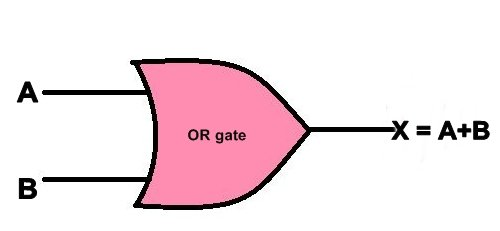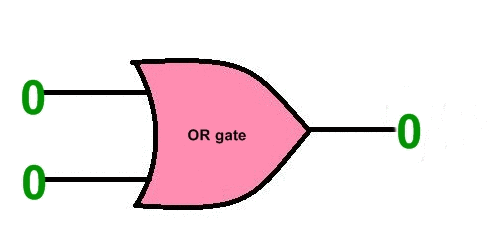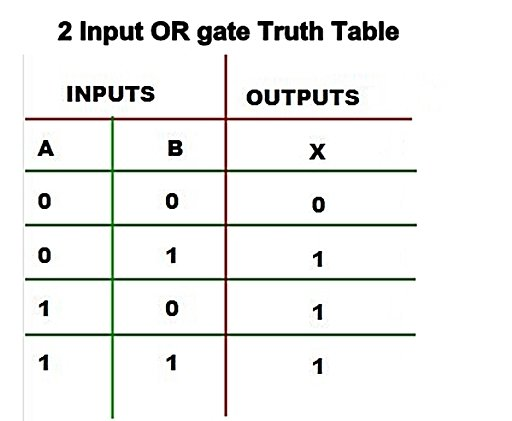### Realization of OR gates can be made using Diodes(Diode Logic) or Transistors(RTL).

In the diode or gate, when both input A and B=0v or LOW , then both diodes D1 And D2 are OFF state because of reverse biasing. So No any current pass Through R and No voltage drop will occur . Then the output X=0v . If both input or any one of both input are +5v . Then the  corresponding diode D1 or D2 is On or both diode D1 and D2 are ON and short circuit occur across them. Therefore the output X is approx to +5v. voltage drop across diode is +5v-0.7v= 4.3v . But this is treated as HIGH or 1.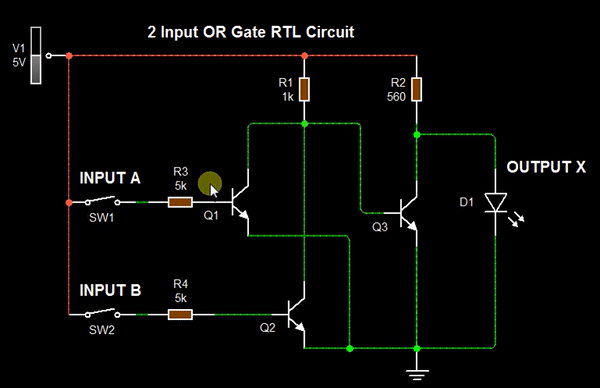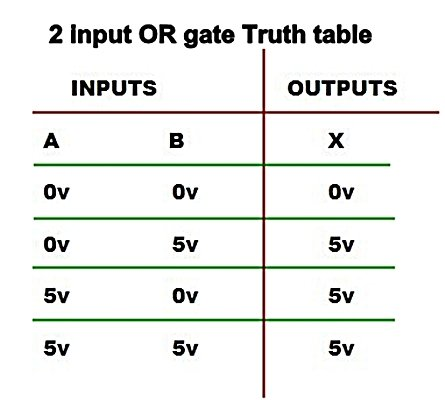In the Transistor (RTL) logic OR gate circuit, When both input A & B are 0v. Transistors Q1 and Q2 are OFF  and Transistor Q3 is ON because of it get Base voltage through Resistor R1. So the output  voltage at Collector pin of Q3 go 0v by dropping of voltage with ground at R2 . If any one of any input A or B is HIGH or 1 then the  corresponding transistors in ON state .  So voltage at the collector of that transistor goes to 0v by voltage drop and there is no any Base voltage at the Q3 and no any voltage drop occurs at R2 therefor the output is HIGH OR 1.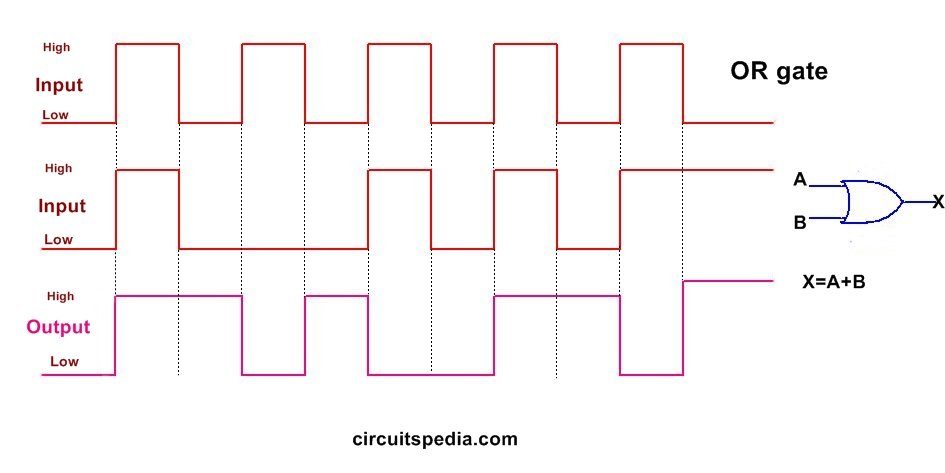## The Universal Gates

Universal gates are defined as Which logic gates can implement any types logic gates . There are two universal  gates NAND and NOR. Both NAND and NOR  gates can perform all the three basic logic functions of AND, OR, NOT.  AOI (AND/OR/INVERT(NOT)) can be converted to NAND logic or NOR logic.

## Types of Universal Gates

### NAND Gate (NOT-AND)

NAND gate is a combination of AND  gate and NOT gate. If the output of AND gate is Inverted then it is called NAND gate. NAND = NOT AND. When AND output is NOTed or Inverted then it is called  NAND gate.The output is 0 only if each inputs is 1 and output is 0 if any one of any input or all inputs are 0. Means when all inputs are 1 then output will 1 otherwise if different combination of input the output is 0. The boolean expression of NAND gate is written as X=

$\overline{A.B.C}$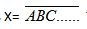This is  read as X=A.B.C….whole bar.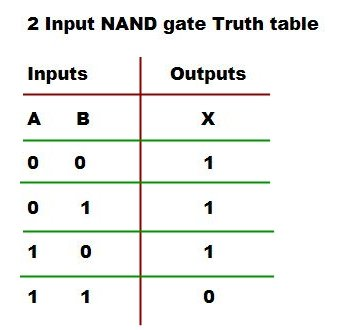#### Realization of NAND gate-

A two input NAND gate can be realized using Diode Transistor Logic. When the input A and B both are HIGH or +5v then both diodes are off and transistor get base voltage through R1 . So the transistor is ON and the output voltage at collector is 0v because of dropped voltage with ground. When Both input A and B are 0v then the both diode are in ON because of forward bias (here 0v means negative supply) . So Base voltage of transistor is 0v. So Transistor is in OFF and then the output is HIGH or approx +5v.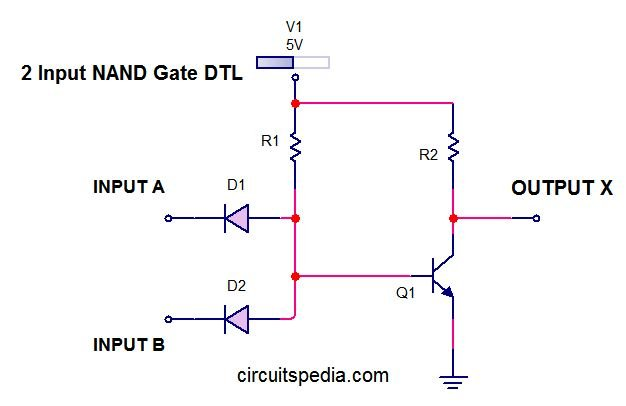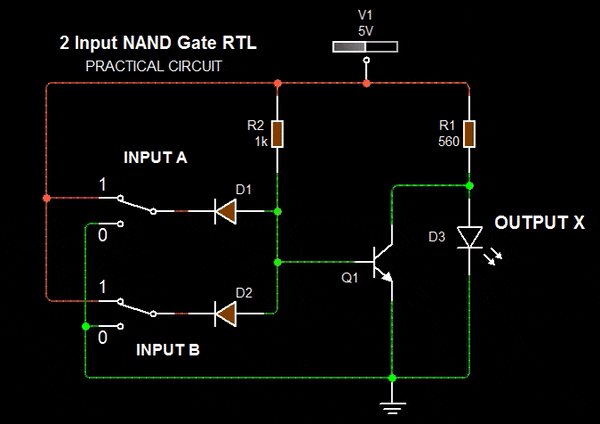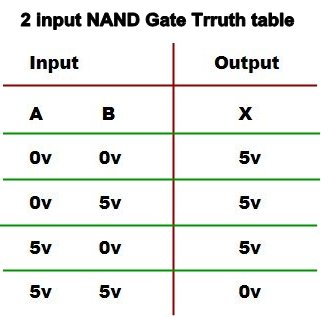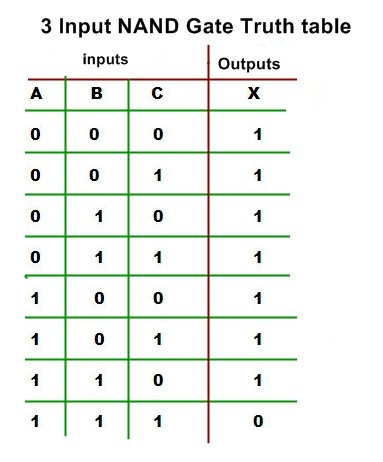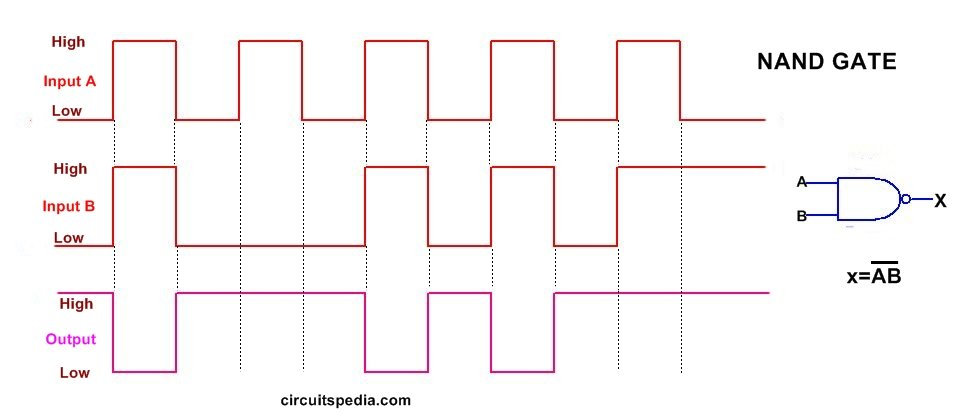### NOR Gate

When the output of OR gate is NOTed or inverted then it is called NOR gate. NOR means NOT OR. NOR gate is the combination of an OR gate and a NOT gate. The output is 1 or HIGH  when only the both input is 0 or LOW. Otherwise output is 0. The boolean expression for the NOR gate is expressed as given below This is read as X is equal to A plus B plus C plus .....whole bar.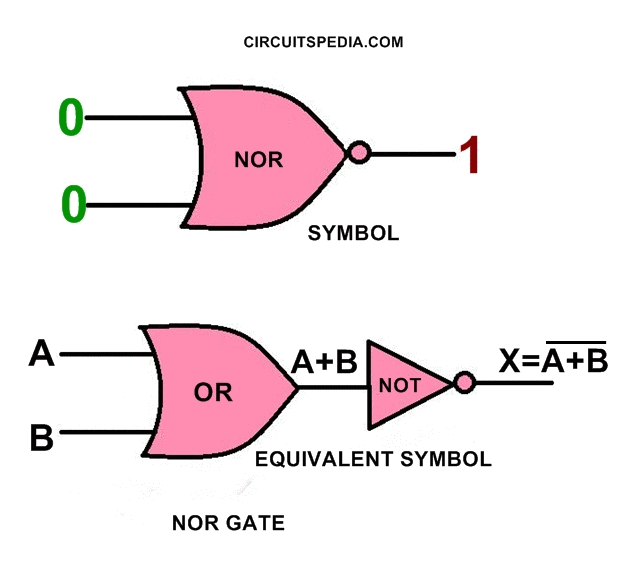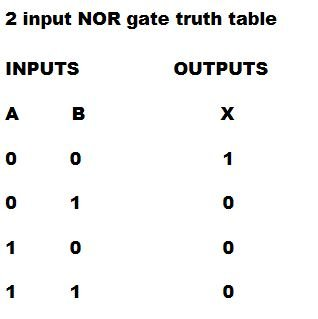### Realization of NOR gate-

Two input RTL(Resistor transistor logic) NOT gate can be realized using two transistors and resistors. When Input A and B both are 0. A=0 and B=0. Then both transistors are OFF because no base voltage get. So no current flows through transistors and no voltage drop occurs. Therefore only current passes through R the output is HIGH.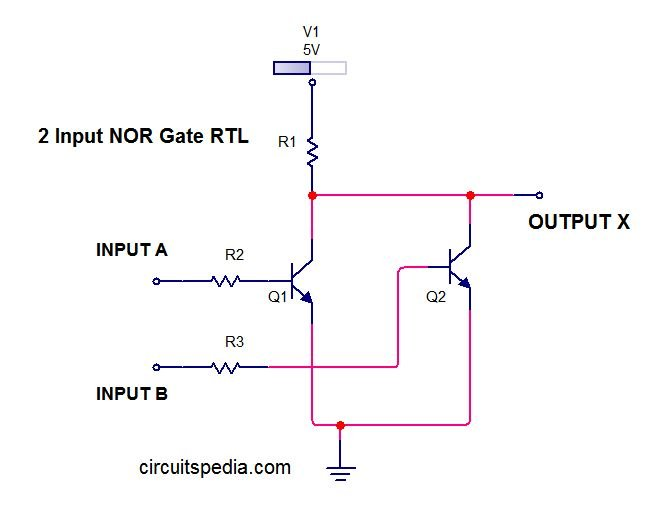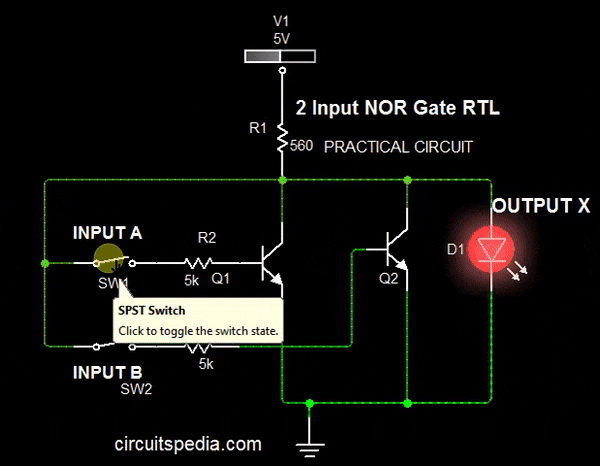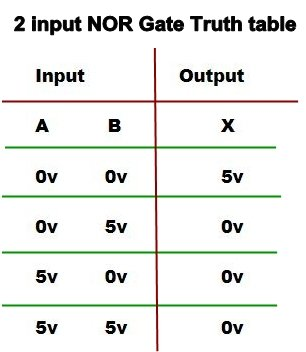###